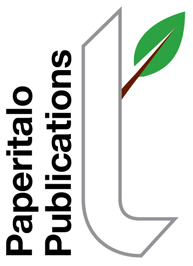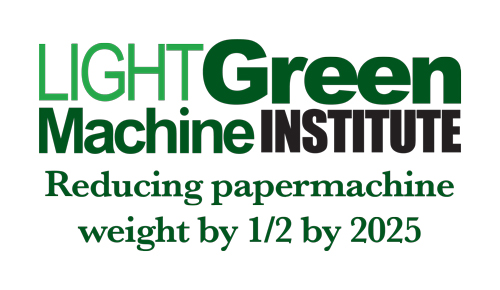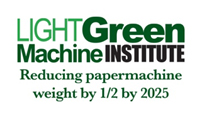The Light Green Machine InstituteAugust 2019: The Optimization Problem--Clues to SolutionBy Jim Thompson   As we talk about optimizing the width/speed of a new paper machine, it may be instructive to start with some theory.   To make it even simpler we'll start with some static theory.   Even simpler still--two-dimensional static theory.   We know that, in two dimensions, the most economical shape, as pertains to boundary versus area encapsulated, is a circle. The formula for the area of a circle is      πr2   If we let r = 3   Then, the area is 28.26   And the circumference is πD = 18.84   So, if we attempt to encapsulate an area of 28.26 in a square instead of a circle, we need a side of length 5.32 to do so [5.322 = 28.3]. But the boundary in this case is 4 x 5.32 = 21.28.   This is 13% more costly than our circle encapsulating the same area [(21.28 - 18.84)/18.84].   If we choose a rectangular shape, it is even worse. For instance, if we choose a rectangle 1 x 28.26, the boundary becomes 1 + 1 + 28.26 + 28.26 = 58.52 , or 213% more costly than the circle and 175% more costly than the square.   Keep in mind, we have stayed with a two-dimensional static problem here. In reality, our machine hall is a three-dimensional building and the web has velocity. Think starting with a sphere (πr3) and thinking about rate of production.   We'll pick up here next month.   By the way, this does not have to be a monologue, chime in!